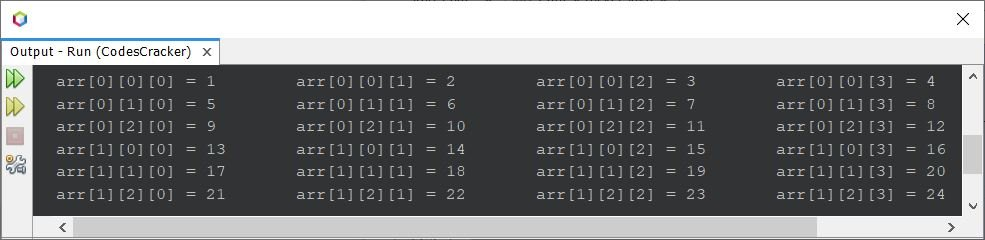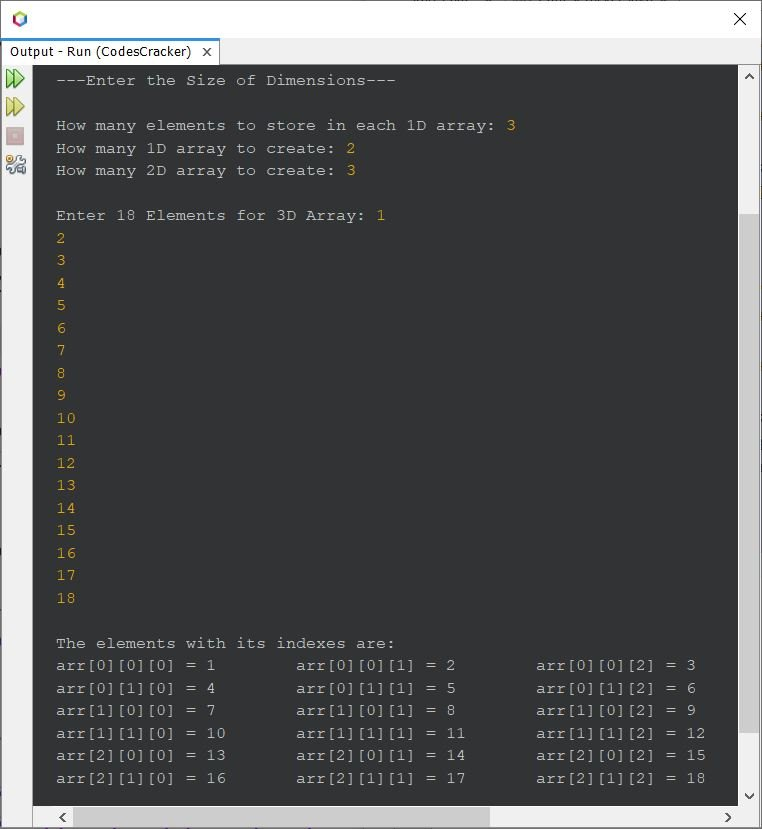# Java Three Dimensional Array Program

A three dimensional array can be said as an array of array of array. For example:

`arr`

is a three dimensional array, where there is a two two-dimensional array, and each two dimensional array contains three one dimensional array, where there are 4 elements in each one dimensional array.

In other words, we can say that the array arr contains 4 elements of 3 array of 2 array. Total of 2*3*4 elements, that is 24 elements. Here is an example:

```public class CodesCracker
{
public static void main(String[] args)
{
int[][][] arr = {
{
{1, 2, 3, 4},{5, 6, 7, 8},{9, 10, 11, 12}
},
{
{13, 14, 15, 16},{17, 18, 19, 20},{21, 22, 23, 24}
}};

for(int i=0; i<2; i++)
{
for(int j=0; j<3; j++)
{
for(int k=0; k<4; k++)
{
System.out.print("arr["+i+"]["+j+"]["+k+"] = " +arr[i][j][k]+"\t");
}
System.out.print("\n");
}
}
}
}```

The snapshot given below shows the sample output produced by above program on three-dimensional array in Java:As you can clearly see from the above program and its sample output, there are two 2D array, where each 2D array is of 3 1D array, where each 1D array is of 4 elements.

Three dimensional array is little complicated concept in programming, that contains three loops. So to print or to initialize elements to a three dimensional array, you need to use three loops. Where the innermost loop makes one dimensional array, the second innermost loop makes two dimensional array, and the outer loop makes finally the three dimensional array.

Here is another program, that allows user to define the size of dimension of three dimensional array, along with its elements:

```import java.util.Scanner;

public class CodesCracker
{
public static void main(String[] args)
{
int one, two, three, i, j, k;
Scanner scan = new Scanner(System.in);

System.out.println("---Enter the Size of Dimensions---\n");
System.out.print("How many elements to store in each 1D array: ");
one = scan.nextInt();
System.out.print("How many 1D array to create: ");
two = scan.nextInt();
System.out.print("How many 2D array to create: ");
three = scan.nextInt();

int[][][] arr = new int[three][two][one];

System.out.print("\nEnter " +(one*two*three)+ " Elements for 3D Array: ");
for(i=0; i<three; i++)
{
for(j=0; j<two; j++)
{
for(k=0; k<one; k++)
arr[i][j][k] = scan.nextInt();
}
}

System.out.println("\nThe elements with its indexes are: ");
for(i=0; i<three; i++)
{
for(j=0; j<two; j++)
{
for(k=0; k<one; k++)
System.out.print("arr["+i+"]["+j+"]["+k+"] = " +arr[i][j][k]+"\t");
System.out.print("\n");
}
}
}
}```

Here is its sample run with user input 3 as number of elements to store in each one dimensional array, 2 as number of one dimensional array to create, 3 as number of two dimensional array to create, and 1, 2, 3, 4, 5, 6, 7, 8, 9, 10, 11, 12, 13, 14, 15, 16, 17, 18 as eighteen elements for the three dimensional array:#### Same Program in Other Languages

Java Online Test

« Previous Program Next Program »